# Engineering Mechanics - KOP: Force and Acceleration

### Exercise :: KOP: Force and Acceleration - General Questions

• KOP: Force and Acceleration - General Questions
11.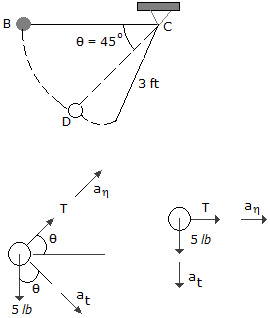The pendulum bob B has a weight of 5 lb and is released from rest in the position shown,=0°. Determine the tension in string BC just after the bob is released,= 0°, and also at the instant the bob reaches point D,= 45°.

 A. T0 = 0, T45 = 10.61 lb B. T0 = 0.1551 lb, T45 = 10.61 lb C. T0 = 0, T45 = 7.07 lb D. T0 = 0.1551 lb, T45 = 7.07 lb

Explanation:

No answer description available for this question. Let us discuss.

12.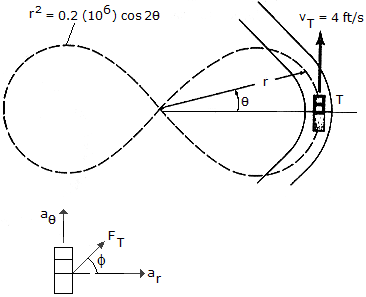A truck T has a weight of 8,000 lb and is traveling along a portion of a road defined by the lemniscate r2 = 0.2(106) cos 2, where r is measured in feet andis in radians. If the truck maintains a constant speed of vr = 4 ft/s, determine the magnitude of the resultant frictional force which must be exerted by all the wheels to maintain the motion when= 0.

 A. F = 29.2 lb B. F = 859 lb C. F = 87.5 lb D. F = 26.7 lb

Explanation:

No answer description available for this question. Let us discuss.

13.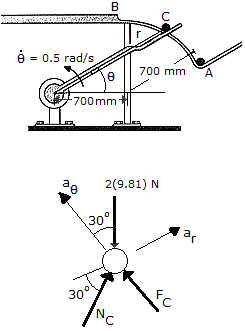A smooth can C, having a mass of 2 kg, is lifted from a feed at A to a ramp at B by a forked rotating rod. If the rod maintains a constant angular motion of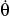= 0.5 rad/s, determine the force which the rod exerts on the can at the instant= 30°. Neglect the effects of friction in the calculation. The ramp from A to B is circular, having a radius of 700 min.

 A. F = 19.62 N B. F = 11.33 N C. F = 10.63 N D. F = 12.03 N

Explanation:

No answer description available for this question. Let us discuss.

14.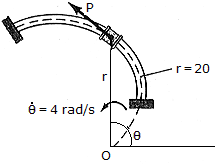The spool, which has a weight of 2lb, slides along the smooth horizontal spiral rod, r = (2) ft, whereis in radians. If its angular rate of rotation is constant and equals= 4 rad/s, determine the tangential force P needed to cause the motion and the normal force that the spool exerts on the rod at the instant= 90°.

 A. P = 0.499 lb, N = 5.03 lb B. P = 5.50 lb, N = 15.65 lb C. P = 3.35 lb, N = 2.43 lb D. P = 1.677 lb, N = 4.77 lb

Explanation:

No answer description available for this question. Let us discuss.

15.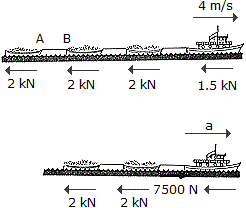Each of the three barges has a mass of 30 Mg, whereas the tugboat has a mass of 12 Mg. As the barges are being pulled forward with a constant velocity of 4 m/s, the tugboat must overcome the frictional resistance of the water, which is 2 kN for each barge and 1.5 kN for the tugboat. If the cable between A and B breaks, determine the acceleration of the tugboat.

 A. a = 0.1667 m/s2 B. a = 0.1042 m/s2 C. a = 0.0278 m/s2 D. a = 0.01961 m/s2